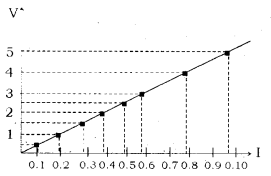# Plot a graph between current (I) and potential difference (V) and determine the resistance (R) of the resistor

The values of current (I) flowing through a given resistor of resistance ( R ), for the corresponding values of potential difference (V) across the resistor are as given below:

V (volts) 0.5 1.0 1.5 2.0 2.5 3.0 4.0 5.0
I (amperes) 0.1 0.2 0.3 0.4 0.5 0.6 0.8 1.0

Plot a graph between current (I) and potential difference (V) and determine the resistance ( R ) of the resistor.

Resistance ( R ) = Slope of line =